> Home

# Structure of sex chromosomes in NGS data

depth-hist.py

```# create a histogram of "samtools depth" outputs
# the output format is:
# #chr  <bin range of [start pos-end pos]>      <# of counted pos>      <total depth>
# bin size is fixed at 1Mb
import sys

def main():
col_chr = 0
col_pos = 1
col_depth = 2
bin_size = 1000000

with sys.stdin as fin:
current_chr = ""
current_start_pos = 0
current_end_pos = 0
total_depth_in_bin = 0
total_count_in_bin = 0
for line in fin:
li = line.rstrip()
ls = li.split("\t")
chrm = ls[col_chr]
pos = int(ls[col_pos])
depth = int(ls[col_depth])
if chrm != current_chr: # chr updated
if current_start_pos != 0:
print "%s\t%d-%d\t%d\t%d" %(current_chr, current_start_pos, current_end_pos, total_count_in_bin, total_depth_in_bin)
current_chr = chrm
current_start_pos = pos
total_depth_in_bin = depth
total_count_in_bin = 1
continue
if pos - current_start_pos + 1 > bin_size: # a bin is full
print "%s\t%d-%d\t%d\t%d" %(current_chr, current_start_pos, current_end_pos, total_count_in_bin, total_depth_in_bin)
current_start_pos = pos
total_depth_in_bin = depth
total_count_in_bin = 1
continue
total_depth_in_bin = total_depth_in_bin + depth
total_count_in_bin = total_count_in_bin + 1
current_end_pos = pos
#end of file
print "%s\t%d-%d\t%d\t%d" %(current_chr, current_start_pos, current_end_pos, total_count_in_bin, total_depth_in_bin)
return 0

if __name__ == '__main__':
main()
```

bin size = 1Mb

```\$ samtools depth -a DRR028634.bam | python ~/depth-hist.py > DRR028634.bam.depth.hist &
```
DRR028634.bam.depth.hist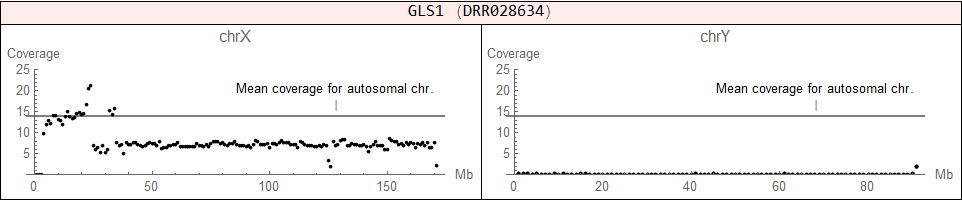♀ (XX), but it has an abnormal structure (X + X fragment)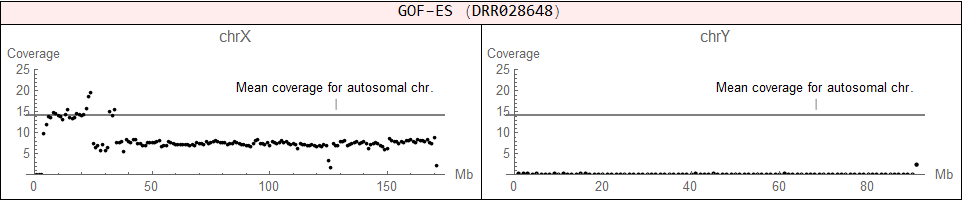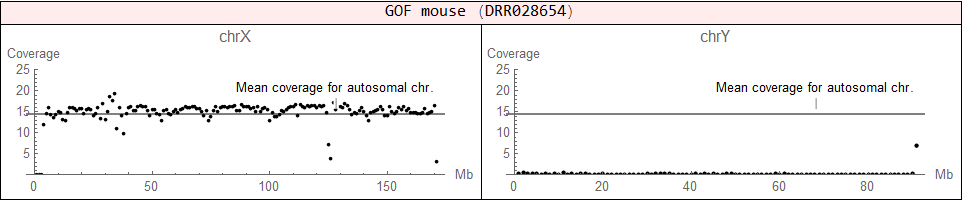♀ (XX)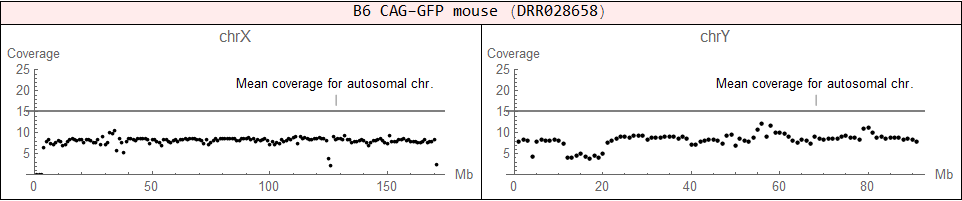♂ (XY)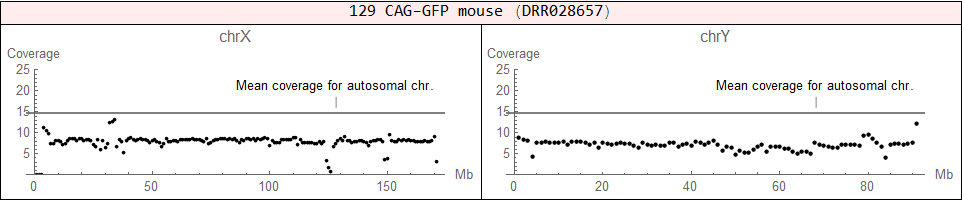♂ (XY)

# Other chromosomal aberrations in NGS data

## Trisomy 8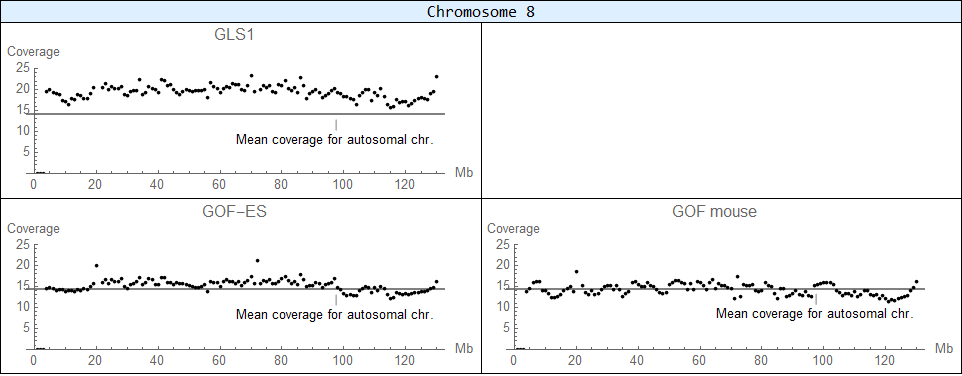Trisomy is detected in GLS1 cells.